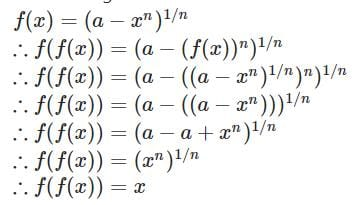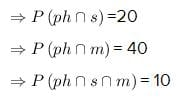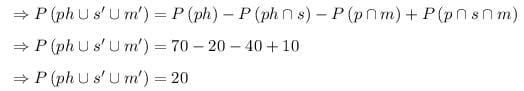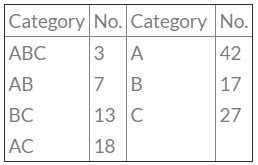# Test: Sets- 3

## 40 Questions MCQ Test Quantitative Aptitude for CA CPT | Test: Sets- 3

Description
Attempt Test: Sets- 3 | 40 questions in 40 minutes | Mock test for CA Foundation preparation | Free important questions MCQ to study Quantitative Aptitude for CA CPT for CA Foundation Exam | Download free PDF with solutions
QUESTION: 1

Solution:
QUESTION: 2

Solution:
QUESTION: 3

### Represent the following sets in set notation: - Set of all alphabets in English language, set of all odd integers less than 25, set of all odd integers, set of positive integers x satisfying the equation x2 + 5x + 7 = 0 :-

Solution:
QUESTION: 4

If ƒ(x) = (a-x)1/n, a > 0 and 'n' is a positive integer, then ƒ(ƒ(x)) = ________________.

Solution:QUESTION: 5

Let A = {1,2,3}, then the relation R  = (1,1), (2,3), (2,2), (3,3), (1,2)} is:

Solution:
QUESTION: 6

The range of {(1,0), (2,0), (3,0), (4,0), (0,0)} is:

Solution:
QUESTION: 7

For a group of 200 persons, 100 are interested in music, 70 in photography and 40 in swimming, further more 40 are interested in both music and photography, 30 in both music and swimming, 20 in photography and swimming and 10 in all the three. How many are interested in photography but not in music and swimming?

Solution:

Let

1. Let P(Ph) is number of person interested in photography .
2. Let P(s) is number of person interested in swimming.
3. Let P(m) is number of person interested in music.

Given:

P(ph)=70 , P(s)=40,P(m)=100The persons who are interested in photography but not in music and swimming is given byThere are 20 persons who are interested in photography but not in music and swimming.

QUESTION: 8

The number of elements in range of constant function is

Solution:
QUESTION: 9

The range of the function f : N → N; f(x) = (-1)x-1, is

Solution:
QUESTION: 10

The set {x | 0 < x < 5} represents the set when x may take integral values only

Solution:
QUESTION: 11

If ƒ(x) = x+2, g(x) = 7*, than g of(x) = __________.

Solution:
QUESTION: 12

Of the 200 candidates who were interviewed for a position at call centre, 100 had a two-wheeler, 70 had a credit card and 140 had a and 140 had a mobile phone, 40 of them had both a two-wheeler and a credit card, 30 had both a credit card and a mobile phone, 60 had both a two-wheeler and a mobile phone, and 10 had all three. How many candidates had none of the three?

Solution:
QUESTION: 13

Which of the following is a subset of set {1, 2, 3, 4}?

Solution:

The subset of set (1, 2, 3, 4} is {1, 2}, {1, 2, 3}, and {1}.

QUESTION: 14

If A = (1,2,3,4,5), B = (2,4) and C = (1,3,5) then (A - C) x B is

Solution:
QUESTION: 15

Let R be a non-empty relation on a collection of sets defined by ARB if and only if A ∩ B = Ø Then (pick the TRUE statement)

Solution:
QUESTION: 16

f(x)  = 3+x , for -3 < x < 0 and 3 - 2x for 0 < x < 3, then value of f(2) will be

Solution:
QUESTION: 17

On the set of lines, being perpendicular is a ___________relation.

Solution:
QUESTION: 18

Out of 1000 student 658 failed in the aggregate, 166 in the aggregate and in group-I 434 in aggregate and in group-II, 372 in group-I, 590 in group-II and 126 in both the groups. How many failed in aggregate but not in group-I and group-II?

Solution:
QUESTION: 19

Out of 1000 student 658 failed in the aggregate, 166 in the aggregate and in group-I 434 in aggregate and in group-II, 372 in group-I, 590 in group-II and 126 in both the groups. Find out how many failed in all the three.

Solution:
QUESTION: 20

For any two sets A and B the set (A∪B')' is Equal to (where' denotes compliment of the set)

Solution:
QUESTION: 21

Out of 1000 student 658 failed in the aggregate, 166 in the aggregate and in group-I 434 in aggregate and in group-II, 372 in group-I, 590 in group-II and 126 in both the groups. How many failed in group-II but not in group-I?

Solution:
QUESTION: 22

Out of 1000 student 658 failed in the aggregate, 166 in the aggregate and in group-I 434 in aggregate and in group-II, 372 in group-I, 590 in group-II and 126 in both the groups. How many failed in group

Solution:
QUESTION: 23

There are 40 students, 30 of them passed in English, 25 of them passed in Maths nd 15 of them passed in both. Assuming that every Student has passed at least in one subject. How many students's passed in English only bot not in Maths.

Solution:
QUESTION: 24

Out of 1000 student 658 failed in the aggregate, 166 in the aggregate and in group-I 434 in aggregate and in group-II, 372 in group-I, 590 in group-II and 126 in both the groups. How many failed in the aggregate but not in group-II?

Solution:
QUESTION: 25

Out of 1000 student 658 failed in the aggregate, 166 in the aggregate and in group-I 434 in aggregate and in group-II, 372 in group-I, 590 in group-II and 126 in both the groups. How many failed in aggregate or group-II but not in group-I?

Solution:
QUESTION: 26

If A = {± 2, ± 3}, B = {1,4,9} and F = {(2,4), (-2,4), (3,9) , (-3,4)} then the 'F' is defined as:

Solution:
QUESTION: 27

Out of total 150 students 45 passed in Accounts 50 in Maths. 30 in Costing 30 in both Account and Maths. 32 in both Maths and Costing 35 in both Accounts and Costing. 25 students passed in all the three subjects. Find the number who passed at least in any one of the subjects.

Solution:
QUESTION: 28

If A has 32 elements B has 42 elements and A∪B has 62 elements find the number of elements in A∩B

Solution:
QUESTION: 29

Out of a total population of 50000 only 28000 read Telegraph and 23000 read Times of India while 4000 read the both. How many do not read any paper?

Solution:
QUESTION: 30

Out of 60 students 25 failed in paper (1), 24 in paper (2), 32 in paper (3), 9 in paper (1), alone 6 in paper (2), alone 5 in papers (2), and (3), and 3 in papers (1), and (2). Find how many passed in all the three papers?

Solution:
QUESTION: 31

On a survey of 100 boys it was found that 50 used white that 50 used white shirt 40 red and 30 blue. 20 were habituated in using both white and red shirts 15 both red and blue shirts and 10 blue and white shirts. Find the number of boys using all the colours.

Solution:
QUESTION: 32

Out 2000 staff 48% preferred coffee 54% tea and 64% cocoa. Of the total 28% used coffee and tea 32% tea and cocoa and 30% coffee and cocoa. Only 6% did none of these. Find the number having tea and cocoa but not coffee.

Solution:
QUESTION: 33

Out 2000 staff 48% preferred coffee 54% tea and 64% cocoa. Of the total 28% used coffee and tea 32% tea and cocoa and 30% coffee and cocoa. Only 6% did none of these. Find the number having only coffee.

Solution:
QUESTION: 34

Out 2000 staff 48% preferred coffee 54% tea and 64% cocoa. Of the total 28% used coffee and tea 32% tea and cocoa and 30% coffee and cocoa. Only 6% did none of these. Find the number having all the three.

Solution:
QUESTION: 35

After qualifying out of 400 professionals, 112 joined industry, 120 started practice and 160 joined as paid assistants. There were 32, who were in both practice and service 40 in both practice and assistantship and 20 in both industry and assistantship. There were 12 who did all the three. Find how many of them did only one of these.

Solution:

Total =  400
Industries Service  S  = 112
Practice  P = 120
Paid Assistant A = 160

P ∩ S = 32
P ∩ A = 40
S ∩ A = 20
P ∩ S ∩ A  = 12

Total = S + P + A - P ∩ S - P ∩ A - S ∩ A + P ∩ S ∩ A + None
400 = 112 + 120 + 160 - 32 - 40 - 20 + 12 + None
None = 88
Person = 400 - 88 = 312
312 - (32 - 12) - (40-12) -(20-12) - 12
= 312 - 20 - 28 - 8 - 12
= 244

QUESTION: 36

Out of 60 students 25 failed in paper (1), 24 in paper (2), 32 in paper (3), 9 in paper (1) alone, 6 in paper (2) alone, 5 in papers (2) and (3) and 3 in papers (1) and (2). Find how many failed in all the three papers.

Solution:
QUESTION: 37

On a survey of 100 boys it was found that 50 used white that 50 used white shirt 40 red and 30 blue. 20 were habituated in using both white and red shirts 15 both red and blue shirts and 10 blue and white shirts. If 10 boys did not use any of the white red or blue colours and 20 boys used all the colours offer your comments.

Solution:
QUESTION: 38

After qualifying out of 400 professionals, 112 joined industry, 120 started practice and 160 joined as paid assistants. There were 32, who were in both practice and service 40 in both practice and assistantship and 20 in both industry and assistantship. There were 12 who did all the three. Find how many could not get any of these.

Solution:
QUESTION: 39

A marketing research team interviews 50 people about their drinking habits of tea coffee or milk or A B C respectively. Following data is obtained but the Manager is not sure whether these are consistent.Solution:
QUESTION: 40

If A = {2, 3}, B = {4, 5}, C = {5, 6} then the set A × (B∩C) is

Solution:Use Code STAYHOME200 and get INR 200 additional OFF Use Coupon Code